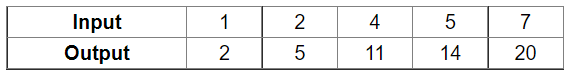# Consider the following input/output table. Draw a line graph for it.

Question:

Consider the following input/output table. Draw a line graph for it.Now, use the graph drawn to predict the outputs for the inputs of 3 and 8.

Solution:

From the graph, we can see that outout for the inputs of 3 and 8 are 8 and 23 respectively.